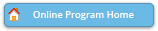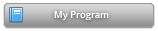#### Abstract Details

 Activity Number: 441 - Recent Advances in Nonparametric Statistics Type: Invited Date/Time: Wednesday, July 31, 2019 : 8:30 AM to 10:20 AM Sponsor: IMS Abstract #300177 Presentation Title: Estimating Rectangular Piecewise Constant Functions in Multiple Dimensions Author(s): Bodhisattva Sen* and Adityanand Guntuboyina and Billy Fang Companies: Columbia University and University of California at Berkeley and University of California at Berkeley Keywords: Completely monotone functions; functions with finite Hardy and Krause variation; nonparametric least squares ; nonnegative least squares problem; parametric rate of convergence Abstract: We consider the problem of estimating rectangular piecewise constant functions in multiple dimensions using the method of least squares. We introduce and study two least squares estimators over: (i) the class of all completely monotone functions (in [0,1]^d), and (ii) the class of functions (in [0,1]^d) with finite Hardy and Krause variation (anchored at 0). The above two classes can be thought of as extensions of the class of all monotone functions and the class of all functions with finite bounded variation on the real line, respectively. We show that the two estimators can be computed by solving a nonnegative least squares problem and a LASSO problem respectively, and furthermore the design matrices in these two finite-dimensional problems are the same. Our main results show that the worst case risk of these estimators do not depend on the dimension d (up to logarithmic factors). Further, these estimators exhibit a parametric (k/n) rate of convergence (up to logarithmic factors) for estimating piecewise constant functions in multiple dimensions, where k is the number of constant "pieces" and n is the sample size.

Authors who are presenting talks have a * after their name.